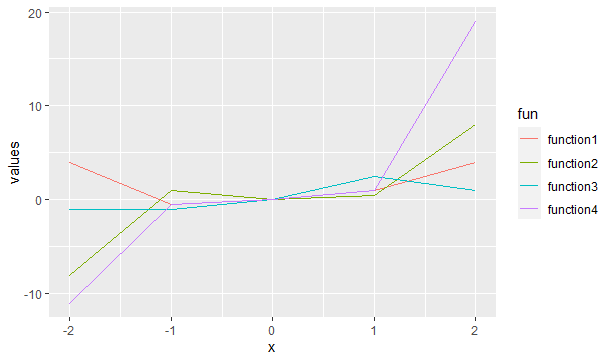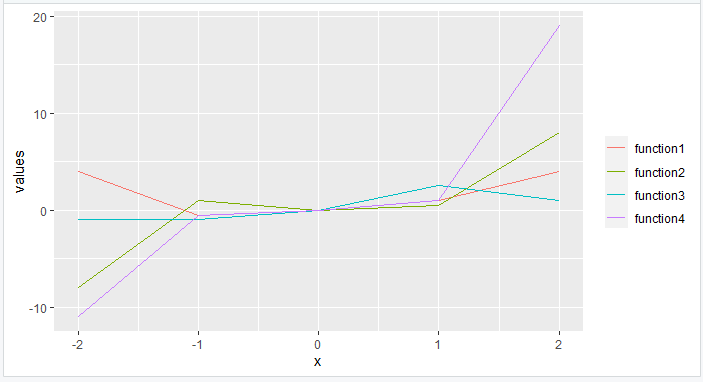Related Articles

# How to remove legend title in R with ggplot2 ?

• Last Updated : 21 Jul, 2021

At times, the legend keys are enough to display what they are supposed to describe. Thus, in such cases, the legend title can be dropped. In this article, we will discuss how the legend title can be removed using ggplot2 in the R programming language.

Let us first draw a regular plot with a legend title:

## R

 `library``(``"ggplot2"``)`` ` `function1<- ``function``(x){x**2}``function2<-``function``(x){x**3}``function3<-``function``(x){x/2}``function4<-``function``(x){2*(x**3)+(x**2)-(x/2)}`` ` `df=``data.frame``(x=-2:2,``              ``values=``c``(``function1``(-2:2),``                       ``function2``(-2:2),``                       ``function3``(-2:2),``                       ``function4``(-2:2)),``              ``fun=``rep``(``c``(``"function1"``,``"function2"``,``                        ``"function3"``,``"function4"``))``)`` ` `ggplot``(df, ``aes``(x, values, col = fun)) + ``geom_line``()`

Output:## Method 1: Using scale_color_discrete()

scale_color_discrete() function deals with legend aesthetics. To remove the title, its name attribute is set to nothing or left black, which makes it not appear in the final plot.

Syntax: scale_color_discrete(name)

Example: Remove legend title using scale_color_discrete().

## R

 `library``(``"ggplot2"``)`` ` `function1<- ``function``(x){x**2}``function2<-``function``(x){x**3}``function3<-``function``(x){x/2}``function4<-``function``(x){2*(x**3)+(x**2)-(x/2)}`` ` `df=``data.frame``(x=-2:2,``              ``values=``c``(``function1``(-2:2),``                       ``function2``(-2:2),``                       ``function3``(-2:2),``                       ``function4``(-2:2)),``              ``fun=``rep``(``c``(``"function1"``,``"function2"``,``                        ``"function3"``,``"function4"``))``)`` ` `ggplot``(df,``aes``(x,values,col=fun))+``geom_line``()``+``scale_color_discrete``(name=``""``)`

Output:## Method 2: Using theme()

theme() function is a powerful way to customize the non-data components of your plots: i.e. titles, labels, fonts, background, gridlines, and legends. To remove legend title, its legend.title attribute is set to element_blank().

Syntax: theme(legend.title= element_blank())

Example: Removing legend title with theme().

## R

 `library``(``"ggplot2"``)`` ` `function1<- ``function``(x){x**2}``function2<-``function``(x){x**3}``function3<-``function``(x){x/2}``function4<-``function``(x){2*(x**3)+(x**2)-(x/2)}`` ` `df=``data.frame``(x=-2:2,``              ``values=``c``(``function1``(-2:2),``                       ``function2``(-2:2),``                       ``function3``(-2:2),``                       ``function4``(-2:2)),``              ``fun=``rep``(``c``(``"function1"``,``"function2"``,``                        ``"function3"``,``"function4"``))``)`` ` `ggplot``(df,``aes``(x,values,col=fun))+``geom_line``()+``  ``theme``(legend.title=``element_blank``())`

Output:My Personal Notes arrow_drop_up